StatLect

# Binomial coefficient

In combinatorics, the binomial coefficient is used to denote the number of possible ways to choose a subset of objects of a given numerosity from a larger set.

It is so called because it can be used to write the coefficients of the expansion of a power of a binomial.## Symbol

The binomial coefficient is denoted by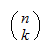and it is read as "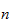choose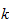" or "over".

## Definition

It is defined as follows: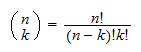where the exclamation mark denotes a factorial.

Reminder: remember the the factorial of a natural numberis equal to the product of all natural numbers less than or equal to: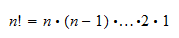and that, by convention,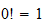.

## Usage in combinatorics

In combinatorics, the binomial coefficient indicates the number of possible combinations ofobjects from.

Example The number of possible ways to choose 2 objects from a set of 5 objects is equal to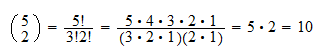## Usage in algebra

In algebra, it is used to expand powers of binomials. According to the binomial theorem,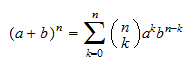Example The third power of a binomial can be expanded as follows: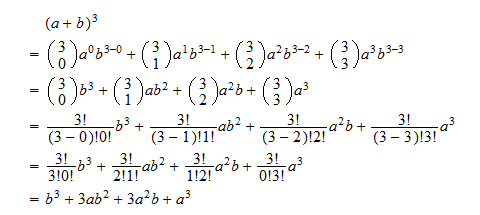## More details

More details can be found in the lecture entitled Combinations, where we explain why combinations can be counted using binomial coefficients, and where we also report some useful recursive formulae that can be used to calculate binomial coefficients.